WINNERS 2019 HISTORY SIXTH GRADE LESSONS ESSAY

The Math Science Nucleus is proud to announce the winners of the Dana Raimondi Math Program Essay Contest on “Why Math is Important.” Competing in 2019 were 300 students from Brier, Blacow,  Durham, Grimmer, Oliveria, and Vallejo Mills, all from Fremont Unified School District. Dr. Karen Anderson, Dana Raimondi and Mrs. Milagro Orantes presented the certificates and prizes.

.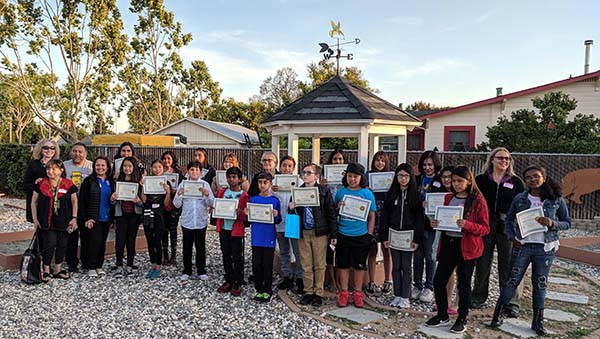Above are the winners both First Place and Honorable Mention of the 2018 Dana Raimondi Math Essay Contest.  Dana Raimondi is center. Below are the First Place winning essays for each of the schools.

Blacow
Elementary

principal:  Mr. Jose Hernandez
Teacher: Janet Calderaro
First: Halley Buenrostro; HM:  Peter Craig
Teacher:  Milagro Orantes

First:  Natalia Campos HM Juan Ceja

Halley Buenrostro
Blacow
Ms. CalderaroMath is very important to science. We use it in physical science. We use it to calculate measurements of objects and their characteristics! As well as to show the relationship between different functions and properties. Arithmetic, algebra and advanced mathematics could be used for complex relationships between properties. I learned a lot of things by Dr. Anderson. She thought me about faces, edges and vertexes for shapes. She thought us how to identify artifacts/ fossils and how to graph them! She has inspired me to learn more about these things and to excel in math and achieve my goal. I enjoyed learning with her.

Natalia Compos

Blacow
Ms. Orantes

You won’t believe how important math to science after this! Science is a subject that needs math to function. Don’t you ever ask yourself, how is math important to science? Well, I have an answer to your question. Of course math is important to science! After all, how do you think medicines are made by that I mean how much of the medicine drugs are added into the prescription. I order to that your need to measure how much of the drug to put, of course you need math in order to measure that. Now that is just one example. Today, you will learning about the many ways math is important to science.

Math is useful tool to simplify science b quantifying of phenomena. In fact, science without mathematics cannot go no further than a humorous oxymoron. You just cannot do physics and chemistry without calculus and statistics, biology without statistics and electrons without complex numbers. In fact, many more things than you can think of is math related to it. For instance, if you are an astronaut and you want to get to the moon, well you sure are not just going to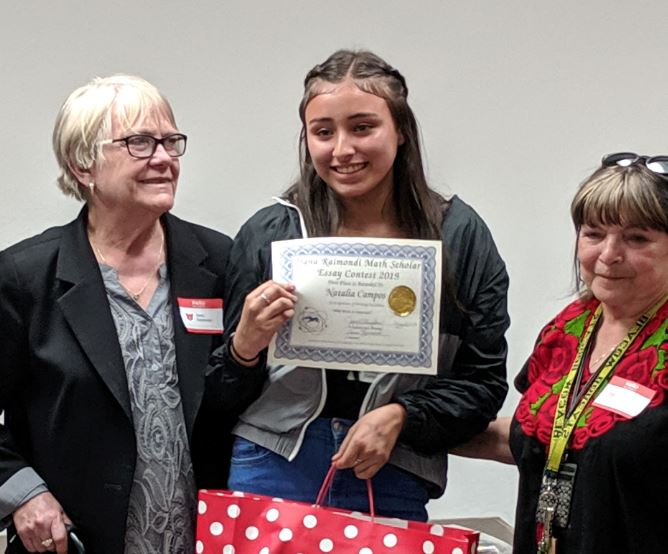go in the middle of nowhere by that I mean, land just anywhere. You need to know how far it is to get to the moon, in order to do that you need math. Angles? Yes, of course how could we forget those angles are science but cannot be identified on what they are without math.

Now there are still many ways that math is related to science, but I will only list a few more. The temperature definitely is another very important thing to science that is math related. Without the temperature, we would not know the weather but in order to know what the weather is going to be like you need numbers which is math. As well for estimating, if you are a paleontologist, the study of fossils, sometimes you come across a fossil and have to estimate its size, as estimation is math. Last, but not least are proportions of liquids, if you are doing a science project or chemistry you will come across maxing liquids together know of course you need to know how much of each liquid you are going to put in each mixture, you obviously not going to mix things out of the blue. In order to do that as well you need math.

In conclusion, I learned a lot more than what I knew about how math is used in science. Now it is your turn to tell me, how do you think math is important to science?Lilia Ten Bosch (Vallejo Mill) Mei Lum (Vallejo Mill) Rahi Vinod (Grimmer)

Brier Elementary
principal:  Mrs. Julie Williams

Teacher:  Karen Narveson
First:  Praghna Palaparthy;  HM:  Mridul Gupta
First: Bhajleen Kaur  HM:  Mehak Maan

First: Diego Gurrola Lopez; HM:  Zina Daliri

Praghna Palaparthy
Brier
Ms. NarvesonMath and science are very two different subjects, right? For example to calculate how the speed, the distance or so on is you need math. You even need math for measuring something or to know how much of something you need. Did you hear about H2O (water). It is made with two H (hydrogen molecules) and one O (oxygen molecule) and add them together, you get H2O, that is a math equation related to science. Most of the scientists were mathematicians too! An example is Galileo Galilei, he had to use math to know how fast gravity pulls. He had to calculate how the earth tilts or that the earth is rotating around the sun. Math is very important to science because of many ways and important to all kinds of sciences. So we know that to learn science you need math too!

Bhajleen Kaur
Brier
Ms. Narveson

Math is used in many ways in science. Astronomers use math to calculate distance of stars and scientists figure out formulas by using math. Why is math so important in science? Science uses numbers every day. For me without math I think there would be no science. For example, if you are making an antidote you need to put the exact measurement to get it just right. If more is poured then instead of making an antidote, you could have made a poison. If math didn’t use math most of the things we own today would not have existed. Math also saves our lives in a way in science. Math and science detect hurricanes, sunamies, earthquakes, volcano eruptions, etc. So math is very important to science in many ways at the point that it can save our lives.

Diego Gurrola Lopez
Brier
Ms. Narveson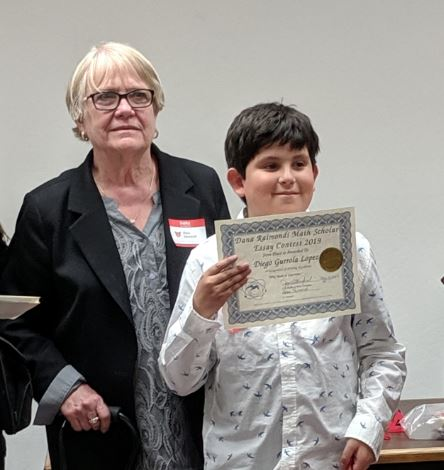Many of the overlooked aspects of math is the data. Data is super important for the world, data proves to the world that yes fetris helps the brain and n fortinite is good (I hate that game!). Without formulas we would never calculate speeds, the area of circle, or the speed of light. Look in a science article, it is hard not to see at least one number. It is like the CPU of a laptop. You need t or else it is wrong and won’t work. It is very good for experiment, too! Ratios can be used to find exact amounts of substance. Overall math is a puzzle in the concept of science.

Durham Elementary
principal:  Mrs. Thersa Bonnacorsi
Teacher:  Joyce Teague
First  Palakbir Hundal ; HM:  Chenyimeng Zhang
Teacher:
Pamela Petrini
First:  Skylar Ruby,  HM:
​Naga Nimma

Palakbir Hundal
Durham
Ms. Teague

Math is important to science

Math and science are two very important branches and they go hand in hand. Science almost revolves around math using equations, formulas, numbers and calculations. Without math science would not even exist! Also, we would be able wearing swimsuits in snow because scientists would not be able to calculate the weather. Our architecture would not be very strong either. We would not know the maximum amount of people a building could hold and if the building was very tall, scientists would not be able to work around major problems like earthquakes.

Neither math nor science can really exist without each other. If someone took equations or formulas out of science, amazing scientists like Albert Einstein would not be able to express their ideas. In conclusion, without math science would crumble in to pieces.

Skylar Ruby
Durham
Mrs. Petrini

Math is very crucial to science. Math can be used to form concepts or find calculations or even group/ organize data used in science. Think about it, science is happening night before our eyes, every day. We use math every day, right? For example, take speed. Math was used to create the concept of speed, speed can lead to movement, which we do every day. To calculate speed, you can either use formula: distance = speed * time or speed = distance / time. That is math. Another example is when we made triangles from lengthy sticks. That is geometry! We were using the triangle enquality property which is when you add up two sides of the triangle (a + b) and is always greater than (c), the last side measure. (a + b > c). That is also math. Another example we did together was the seashell lab. We counted each seashell and organized each shell by type. We filled in information about each shell, and saw what percent of the shells were classified to be out of 100. We made each percent a decimal, fraction and added all the percent to show the total. This is definitely math by organizing concepts. My last example of math in science is when we partitioned the archeology site in to four quadrants. We organized data and measured each bone in inches. As tou can see, all these different sciences include math in some way, size, shape or form. Math is used in almost every science whether it has to do with concepts and formulas to evaluate, or organize data. Math and science are commonly used together. After all, STEM = science, technology, engineering and ……. Math! Everything you see can be broken down into shapes, which is also math. As you can see this is how math is very important to science.

Grimmer Elementary
principal:  Ms. Judy Nye

Teacher:  Teresa Silva
First: Karina Ramirez; HM Jasmine Yolangco
Teacher:
Rina Ortega
First: Hope Ott, HM:  Mia Fiorelli

Karina Ramirez
Grimmer
Ms. Silva

Math is important to Science in many ways. Math helps Scientists calculate things and measure things when they are doing an experiment. They also use math to record their evidence and data. Scientists and people use Math in Chemistry, Physics and Astronomy.

Many people use math in Astronomy. They use the math to calculate the distance of planets. They also use math when they are looking at objects in the sky. They use numbers to count the electrons of the objects and the size. Scientists also use math to count light years. Also they use math to measure the sizes of planets and use it to collect their evidence.

Math is also used in Chemistry. The math helps people make equations. Math also helps people analyze things and make theories about them. They also use the math when they are measuring items or when they count many atoms or cells are inside something. Math is also useful when they are adding compounds together helps them keep track.

Math is also very useful in Physics. Math helps the people figure out the time while doing Physics. When they are studying force and energy math helps them with their job. Also with motion. Scientists say without math, Physics would be more difficult.

As you can see, math is useful in many ways. Math is used in Chemistry, Physics and more.

Hope Ott
Grimmer
Ms. Ortega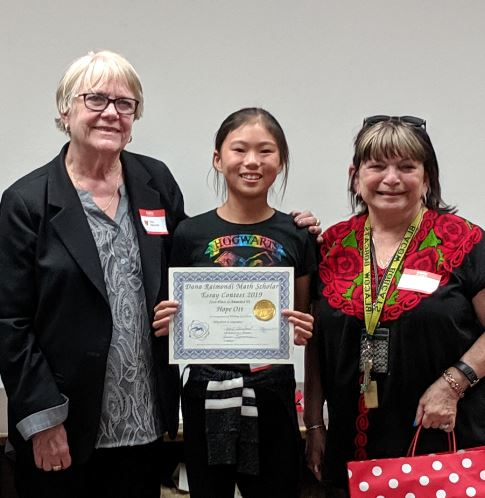Math and Science are both very important parts of our life. Both math and Science are linked and depend on each other. Math is important to Science because it is basically a language of numbers that can help us keep track of items or height, when tyring to conduct experiments. It can also help us calculate better to understand things better.

Math is useful as a language to keep track of things and be able to calculate to comprehend Science better. An example of using math to keep track of things could be in the morning when you check the weather it shows the temperature in numbers. An example of using math to calculate scientific research when scientists measure wind speed by using division and much more.

Because math and science are so important in our lives, it is important to know how they are connected. Being able to keep track of things better and being able to calculate scientific research are benefits of math to science. They depend on each other and help make the world what it is.

Oliveria Elementary
principal:  Mrs. Linda Anderson

Teacher:  Heather Tilghman
First: Rehan Anosh HM:
Viveka Sankaranarayanan Teacher:  Shantelle Johnson Steele
First:  Sophia Perry, HM Angad Gill
Teacher:  Jason Hahn
First:  Rachelle Jimenez HM: Aadam Tayeb
Teacher:  Michelle Lemaster
First: Kyli Agno HM: Kiesha White

Rachelle Jimenez
Oliveira
Mr. Hahn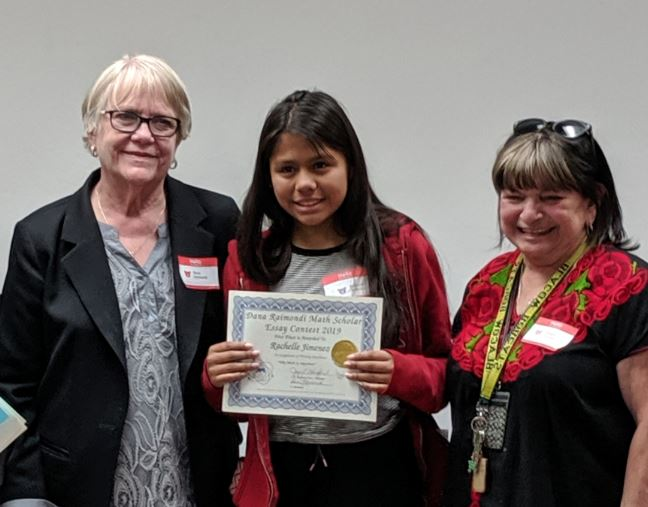Why is math important to Science, you might ask? Math is important to Science because you could record data. This is important because without being able to record data you would not know how long or the temperature or whatever. Another reason why math is important to Science is that you could find the mass or matter or the object. Without knowing how to find the matter you won’t be able to do experiment like how much water rises with a tiny rubber ball. The last why math is important with Science is because of all of the charts. The charts help us to record data and analyze what was happening. In conclusion, there are many reasons why math is important to Science.

Kylie Agno
Oliveira
Mrs. Lemaster

Math is important to Science for many reasons. For instance, it helps collect data or information for experiments. Determine the age of many different types. And so on. We can use math for Science on measurements, including testing. Math is important for many things, including Science. Helps with hypothesis and calculations. And Science is to understand more about the real world. Nature, space, oceans, our states/ countries, etc. Without math, we can’t figure out how much items in a bag costs. Without Science, we can’t figure out new discoveries. That is why math and Science really make a good combination. It helps for many good reasons.

Rehan Anosh
Oliveira
Ms. Tilghman

Math is important to Science because it keeps track of the growing population of animals, mammals, fish and bugs. It also helps us in one of our most ambitious science, rocket science. Even though you may think that math is not needed it is a large part of rocket science. Another science that needs math is wavelength. Wavelength measures how long, wide and tall it is. Without it, we would not know what the ozone layer protects us from. Without it, we even would not go to the moon, or mars, and Venus. Without math, we could not discover planets we even could not see. Math like controls us back in the day, you would not need math at all, people eyeballed what they needed. In our world math helped us to evolve to the humans we are today.

Vallejo Mill Elementary
Principal  Rosalinda Cuebas Carrero
Teacher:  Julie Chen
First: Rahi Vinod,  HM: Zayan Ahmed
Teacher:  Nancy Finney
First:  Jordon Brown; HM Kaitlyn Lafrentz
Teacher: Ana Hoppenbrouwers
First:  Lilia Ten Bosch   HM: Sarah Tran

Jordan Brown
Vallejo Mill
Ms. Finney

Science or Math?

Have you ever wondered how humans evolved so much? Well, the answer is math! Math is in everything, not just a textbook. Look around, money, chemistry, physics, do you see numbers, measurements, or data analysis? All those things use math to help us utilize and understand them. The following shows examples at how we use math in the real world of science.

Math is the way almost everyone has fun. Do you play sports? Well, to make the ball, engineers use measurements and machines to mold the ball into that perfect shape. Do you live in a house of apartment? To make those buildings, they use something called architecture to design floor plans and walls. After the designs are made in the construction phase, they have to make sure that every piece is even so that nothing collapses. This is possible through measurement and geometry. While we are on the subject of houses, think of electricity, if electricians don’t use correct calculations we may leaveroom for electricity to escape or not fully change our technology. Scientists do studies and track data using statistics like mean, median, mode and range. They analyze the data to find answers to their hypothesis and predict outcomes.

The biggest connection between math and science is coding in my opinion. Do you play video games? How do you think these are created? The answer is math. Coding is what we use for all and any tech, without it you couldn’t have Youtube, Netflix or cable. Technology is science that almost everyone uses every day, from iphones to a clock, it is all science obtained through math. Another example of how math relates to science is that paleontologists use carbon dating to see how old artifacts are and determine what civilization that it might have come from. If we can now when an artifact is from, we can create a timeline to help us understand the importance to the age it was. Chemistry is another example of math in Science because without the correct measurements, the compound created would not have the right outcome. Also without chemistry, we would not have medicines for many diseases and sickness, and we would not have chemistry without math. In physics we use velocity to measure forces such as gravity. We take measurements of distance and speed to see how different forces here no earth.

Is math the cornerstone of science? Well, based off my examples above, I think that I have proven that without math many scientific methods would not be possible, useful, or helpful. What do you think?

Rahi Vinod
Vallejo Mill
Ms. Chen

Math is also Science!

Some people think that math and science are two separate subjects. That is true, but did you know people use math for science. Some people think that math is not important to science, but math is super important to science because we use math in science for lots of things. We use math for, cooking. When you are making a cake you need to see how much milk you need, so you use a measuring cup. You use a measuring cup for everything, for water, flour, sugar and milk. Another source of math you can find in science is a thermometer. You use a thermometer for checking some ones temperature, how high it is outside. We use math for lots of stuff. This is also math, and we use that for science as well. We can check time by clocks and watches. We can check how many days it took for the cherry tree to grow cherries. We use measuring like measuring how deep swimming pool is, or measuring how many light years it takes for the earth to orbit. Now, you see that math is important for science. Math and science are like friends!

Lilia Ten Bosch
Vallejo Mill
Ms. Hoppenbrouwers

Science is needed in math because we need to measure formulas. For example, how much venom can kill a human being or monkey. Science would be even more complicated without math. Human beings would not know what to do and be very confused. We also need algorithms (equations) for formulas of chemicals and mysterious liquids or solids discovered. We would also need math if anyone would leave a chemical to sit and process or mix. Or of somebody were to let a fruit out for a certain amount of time to see how much mold would form. After all of my examples you can see that without math there would be no science.

[HOME]  [Raimondi Home]